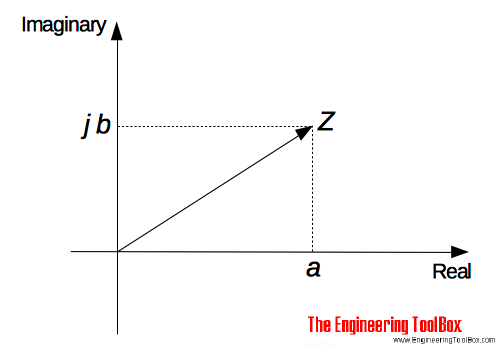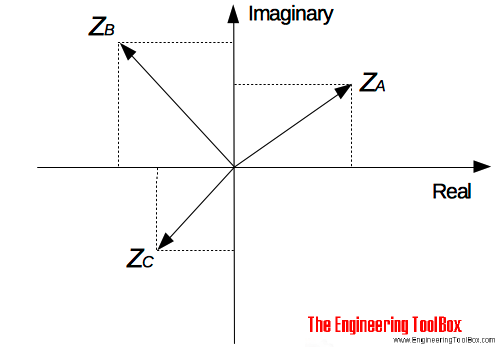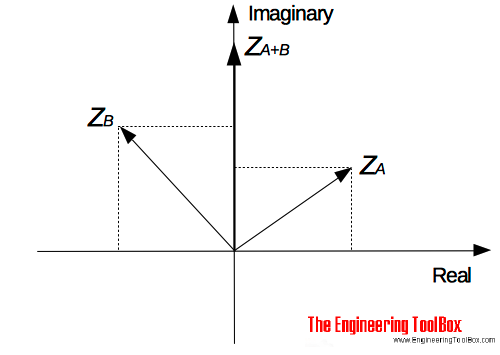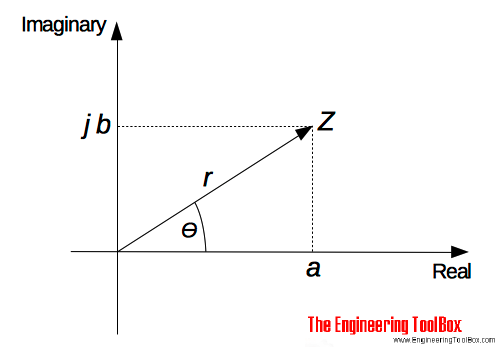Engineering ToolBox - Resources, Tools and Basic Information for Engineering and Design of Technical Applications!

# Complex Numbers

## Complex numbers are used in alternating current theory and mechanical vector analysis.

There are two main forms of complex numbers

• Cartesian
• Polar

### Complex numbers on the Cartesian form

A complex number consists of a real part and an imaginary part and can be expressed on the Cartesian form as

Z = a + j b                         (1)

where

Z = complex number

a = real part

j b = imaginary part (it is common to use i instead of j)

A complex number can be represented in a Cartesian axis diagram with an real and an imaginary axis - also called the Argand diagram:#### Example - Complex numbers on the Cartesian form

The complex numbers

ZA = 3 + j 2                              (2a)

ZB = -3 + j 3                             (2b)

ZC = -2 - j 2                             (2c)

can be represented in the Argand diagram:#### Addition and Subtraction of Complex numbers

Complex numbers are added/subtracted by adding/subtracting the separately the real parts and the imaginary parts of the number.

##### Example - Adding two Complex numbers

ZA = 3 + j 2

ZB = -3 + j 3

Z(A+B) = (3 + (-3)) + (j 2 + j 3)

= j 5### Complex numbers on the Polar form

A complex number on the polar form can be expressed as

Z = r (cosθ + j sinθ)                   (3)

where

r = modulus (or magnitude) of Z - and is written as "mod Z" or |Z|

θ = argument (or amplitude) of Z - and is written as "arg Z"r can be determined using Pythagoras' theorem

r = (a2 + b2)1/2                          (4)

θ can be determined by trigonometry

θ = tan-1(b / a)                          (5)

(3) can also be expressed as

Z = r ej θ                    (6)

As we can se from (1), (3) and (6) - a complex number can be written in three different ways.

#### Example - Complex number on the Polar form

The complex number

Za = 3 + j 2

can be expressed on the polar form by calculating the modulus and the argument.

The "modulus" can be calculated by using eq. (4):

r = (32 + 22)1/2

=  3.606

The "argument" can be calculated by using eq. (5):

θ = tan-1(2 / 3)

= 33.69o

The complex number on polar form (3):

Za = 3.606 (cos(33.69) + j sin(33.69))

Or alternatively (6)

Za = 3.606 ej 33.69

### Adding or Subtraction of Complex Numbers

Za = a + j b

Zb = c + j d

Za + Zb = (a + j b) + (c + j d)

= (a + c) + j(b + d)                            (6)

or alternative

Za = ra (cosθa + j sinθa)

Zb = rb (cosθb + j sinθb)

Za + Zbra (cosθa + j sinθa) + rb (cosθb + j sinθb)

= (ra cosθa + rb cosθb) + j (ra sinθarb sinθb)                            (6b)

or alternatively

Za = ra ejθa

Zb rb ejθb

Za + Zb = ra ejθa + rb ejθb

= (ra cosθa + rb cosθb) + j (ra sinθarb sinθb)                            (6c)

##### Example - Adding Complex Numbers

Za = 3 + j 2

Zb = 5 - j 4

Za + Zb = (3 + j 2) + (5 - j 4)

= (3 + 5) + j(2 + (-4))

= 8 - j 2

##### Example - Adding Complex Numbers

Za = 3 (cos 35 + j sin 35)

Zb = 2 (cos 15 + j sin 15)

Za + Zb = (3 cos 35+ 2 cos 15) + j (3 sin 35 +  2 sin 15)

= 4.38 - j 2.2

#### Subtracting Complex Numbers

Za = a + j b

Zb = c + j d

Za - Zb = (a + j b) - (c + j d)

= (a - c) + j(b - d)                           (7)

##### Example - Subtracting Complex Numbers

Za = 3 (cos 35 + j sin 35)

Zb = 2 (cos 15 + j sin 15)

Za - Zb = 3 (cos 35 + j sin 35) - 2 (cos 15 + j sin 15)

= (3 cos 35 - 2 cos 15) + j (3 sin 35 - 2 sin 15)

=  0.52 + j 1.2

### Multiplication of Complex Numbers

Za = a + j b

Zb = c + j d

Za Zb = (a + j b) (c + j d)

= a c + a (j d) + (j b) c + (j b) (j d)

= a c + j a d + j b c + j2 b d                           (8)

Since j2 = -1  - (8) can be transformed to

Za Zb = (a + j b) (c + j d)

= (a c - b d) + j (a d + b c)                          (8b)

##### Example - Multiplying Complex Numbers

Za3 + j 2

Zb5 - j 4

Za Zb = (3 + j 2) (5 - j 4)

= (3 5 - 2 (-4)) + j(3 (-4) + 2 5)

= 23 - j 2

### Complex Conjugate

The complex conjugate of (a + jb) is (a - jb).

Multiplying a complex number with its complex conjugate results in a real number like

Za = a + jb

Za* = a - jb

Za Za* = (a + jb) (a - jb)

= a2 - j a b + j a b - j2 b2

= a2 - (- b2)

= a2 + b2                                 (9)

##### Example - Multiplying a Complex Number with its Conjugate

Za3 + j 2

Za*3 - j 2

Za Za* = (3 + j 2) (3 - j 2)

= 32 + 22

= 13

### Division of Complex Numbers

Division of complex numbers can be done with the help of the denominators conjugate:

Za = a + jb

Zbc + j d

Za / Zb = (a + j b) / (c + j d)

= ((a + j b) / (c + j d)) ((c - j d) / (c - j d))

= (a c +  j a d + j b c + j2 b d) / (c2 + d2)                                  (10)

Multiplying both the nominator and the denominator with the conjugate of the denominator is called rationalizing.

## Related Topics

• ### Mathematics

Mathematical rules and laws - numbers, areas, volumes, exponents, trigonometric functions and more.

## Related Documents

• ### AC - Active, Reactive and Apparent Power

Real, imaginary and apparent power in AC circuits.
• ### Algebraic Expressions

Principal algebraic expressions formulas.
• ### Decimal System Prefixes

Prefix names used for multiples and submultiples units.
• ### Designation of Large Numbers

Designation of large number in US vs. other countries.
• ### Differential Calculus

Derivatives and differentiation expressions.
• ### Fractions

Law of fractions
• ### Hyperbolic Functions

Exponential functions related to the hyperbola.
• ### Numbers - Square, Cube, Square Root and Cubic Root Calculator

Calculate square, cube, square root and cubic root. Values tabulated for numbers ranging 1 to 100.
• ### Polar vs. Cartesian Coordinates

Convert between Cartesian and Polar coordinates.

Radian is the SI unit of angle. Convert between degrees and radians. Calculate angular velocity.
• ### Roman Numerals

Roman numerals are a combinations of seven letters.
• ### Triangle

Triangle analytical geometry.
• ### Trigonometric Functions

Sine, cosine and tangent - the natural trigonometric functions.

Online vector calculator - add vectors with different magnitude and direction - like forces, velocities and more.

## Engineering ToolBox - SketchUp Extension - Online 3D modeling!

Add standard and customized parametric components - like flange beams, lumbers, piping, stairs and more - to your Sketchup model with the Engineering ToolBox - SketchUp Extension - enabled for use with older versions of the amazing SketchUp Make and the newer "up to date" SketchUp Pro . Add the Engineering ToolBox extension to your SketchUp Make/Pro from the Extension Warehouse !

We don't collect information from our users. More about

## Citation

• The Engineering ToolBox (2016). Complex Numbers. [online] Available at: https://www.engineeringtoolbox.com/complex-numbers-d_1921.html [Accessed Day Month Year].

Modify the access date according your visit.

9.27.11